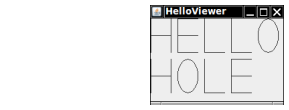Write methods

• public static void drawH(Graphics2D g2, Point2D.Double p);

• public static void drawE(Graphics2D g2, Point2D.Double p);

• public static void drawL(Graphics2D g2, Point2D.Double p);

• public static void drawO(Graphics2D g2, Point2D.Double p);

that show the letters H, E, L, O on the graphics window, where the point p is the top-left corner of the letter. Then call the methods to draw the words “HELLO” and “HOLE” on the graphics display. Draw lines and ellipses. Do not use the drawString method. Do not use System.out.

Here is a sample program output:Complete the following file:

### Letters.java

Use the following files:

HelloViewer.java

```import javax.swing.*;

/**
This program shows the message "HELLO".
*/
public class HelloViewer
{
public static void main(String[] args)
{
JFrame frame = new JFrame();

final int FRAME_WIDTH = 200;
final int FRAME_HEIGHT = 150;

frame.setSize(FRAME_WIDTH, FRAME_HEIGHT);
frame.setTitle("HelloViewer");
frame.setDefaultCloseOperation(JFrame.EXIT_ON_CLOSE);

LettersComponent component = new LettersComponent();

frame.setVisible(true);
}
}
```

LettersComponent.java

```import javax.swing.JComponent;
import java.awt.Graphics;
import java.awt.Graphics2D;
import java.awt.Rectangle;
import java.awt.geom.Point2D;

public class LettersComponent extends JComponent
{
public void paintComponent(Graphics g)
{
final int WIDTH = 30;
final int HEIGHT = 50;
final int SPACING = 10;

Graphics2D g2 = (Graphics2D) g;

Rectangle bounds = new Rectangle(0, 0, WIDTH, HEIGHT);

Letters.drawH(g2, bounds);
bounds.translate(WIDTH + SPACING, 0);

Letters.drawE(g2, bounds);
bounds.translate(WIDTH + SPACING, 0);

Letters.drawL(g2, bounds);
bounds.translate(WIDTH + SPACING, 0);

Letters.drawL(g2, bounds);
bounds.translate(WIDTH + SPACING, 0);

Letters.drawO(g2, bounds);
bounds = new Rectangle(0, HEIGHT + SPACING, WIDTH, HEIGHT);

Letters.drawH(g2, bounds);
bounds.translate(WIDTH + SPACING, 0);

Letters.drawO(g2, bounds);
bounds.translate(WIDTH + SPACING, 0);

Letters.drawL(g2, bounds);
bounds.translate(WIDTH + SPACING, 0);

Letters.drawE(g2, bounds);
}
}
```